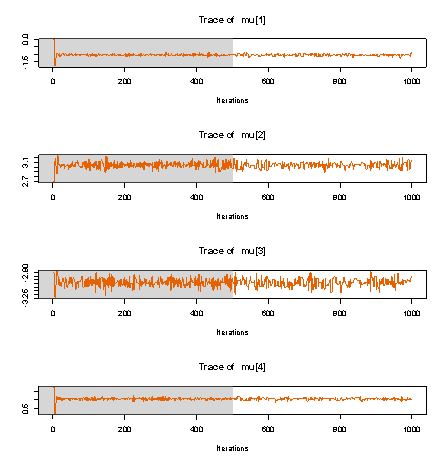Sep 30

# Review of STAN: off-the-shelf Hamiltonian MCMC

Recently, some folks at Andrew Gelman’s research lab have released a new and exciting inference package called STAN.  STAN is designed to do MCMC inference “off-the-shelf”, given just observed data and a BUGS-like definition of the probabilistic model.  I’ve played around with STAN in some detail, and my high-level review is summarized here

Good:

• Installation is involved but straight-forward and quite well-documented
• STAN has an active and enthusiast developer team. Ask a question on the Google group, and you’ll often get a same day (or even same hour) response.
• STAN does Hamiltonian MCMC via automatic differentiation and principled automatic tuning, so you (1) don’t need to compute any gradients analytically, and (2) don’t need to set any parameters manually!
• STAN is also wicked fast on some toy examples it was designed for.

Caveats:

• STAN (version 1.0) does not support *any* sampling of discrete variables. You’ll need to invest human time in marginalizing out discrete variables, esp. for common machine learning approaches like mixture models.
• Vectorizing the model definition can work wonders, but requires some detailed human knowledge of STAN’s inner guts.
• The type system is a bit confusing. For example, to enforce that a variable must be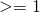:
• This is legal: real<lower=1> x;
• This isn’t: vector<lower=1> xvec;
• Some language features I expected were mysteriously lacking.
• cannot slice-index variables (e.g. MyMatrix[ 1:5,:] )
• cannot transpose matrices

Punchline: I don’t think STAN is quite ready to be used by Machine Learning researchers as a black-box tool to prototype models.  It lacks fine control over when to do discrete vs. continuous updates, and thus scales poorly to moderately sized dataset (it took *minutes* to run 5 iterations on an LDA topic model with just 100 documents and 7000 words).  However, I do believe that the automatic approach to Hamiltonian MCMC is sensible, and hopefully down the road this package might be more viable.

## Example 1: Mixture of Gaussians

To get my feet wet with STAN, I first attempted a standard Mixture of Gaussians model.Mixture Probabilities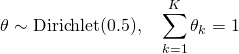Mixture Components (Locations of the Gaussian means):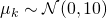data points (scalars)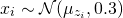The inference problem is to find the hidden mixture parametersand data-specific assignments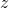given observed data.  We’ll assume fixed variance parameters throughout this example, though STAN certainly supports inferring these as well.

### First STAN Program (WRONG)

Here’s my first attempt at a STAN model specification:

data {
int K;
int N;
real x[N];
}

parameters {
simplex[K] theta;
real mu[K];
int z[N];
}

model {
real ps[K];
for (k in 1:K) {
mu[k] ~ normal( 0, 10 );
}
for (n in 1:N) {
z[n] ~ categorical( theta );
x[n] ~ normal( mu[ 1+z[n] ], .3 );
}
}

Unfortunately, this specification *does not work* in practice! The problem is that STAN cannot handle the unknownsince they are not continuous. The solution is to integrate out these discretevariables.

### Correct STAN Program

For our mixture model, this marginalization can be done easily. We just express the distribution over theobservations purely in terms of continuous, global parameters: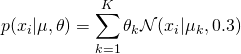Of course, this new representation defines the same *marginal distribution* for our variables of interest, but encoding this new view of the model into STAN directly requires some trickery. Since this new view of the distribution ofisn’t expressed by standard density functions, we’ll just tell STAN directly how to compute the log posterior probability lp__ of the data given the model:

model {
real logps[K];
for (k in 1:K) {
mu[k] ~ normal( 0, 10 );
}
for (n in 1:N) {
for (k in 1:K) {
logps[k] <- log(theta[k]) + normal_log( x[n], mu[k], .3);
}
lp__ <- lp__ + log_sum_exp( logps );
}
}

This improved version NormalMixCont.stan works just fine. I ran it on this sample univariate dataset, where 100 data pointscome from one of four well-separated modes located at (-3, -1, 1, +3). Here’s a histogram view of this data: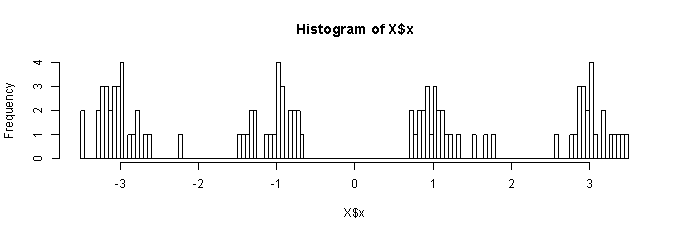Now, we’ll just open up R with rstan properly installed and run inference on this data!

> library(rstan)
> traceplot(F, 'mu') # plot mixture locs
Here’s that final traceplot (showing the learned cluster locations). We can see that STAN has easily found the four true locations (-3, -1, 1, 3):